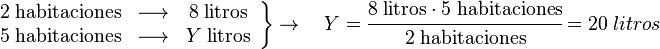Posted on

# The Rule of Three in MathematicsThe Rule of Three is a Mathematical Rule that allows you to solve problems based on proportions. By having three numbers: a, b, c, such that, ( a / b = c / x), (i.e., a: b :: c: x ) you can calculate the unknown number. The Rule of Three Calculator uses the Rule of Three method to calculate the unknown value immediately based on the proportion between two numbers and the third number.

The working of the Rule of Three Calculator can be expressed as follows:Here, there are two values:  A and B and a value of X. In order to find out the value of Y, the Rule of Three tells me that B is related to A and that Y is related to X.

## How does the Rule of Three Calculator work?

Simply fill in the fields of the Maths Calculator with the values you want to work out (Value A, Value B and Value X), press the calculate button and the Rule of Three Calculator will immediately display the missing value of Y.

## An example of the Rule of Three:

An example that you would use to explain the rule of three is as follows:

If I have 8 litres of paint for 2 bedrooms, how many litres of paint would I need for 5 bedrooms?

In this case, the two values of A and B are known, A) 2 bedrooms and B) 8 litres. The value of X is also known ( 5 bedrooms) and the missing value is that of Y ( the number of litres) So: